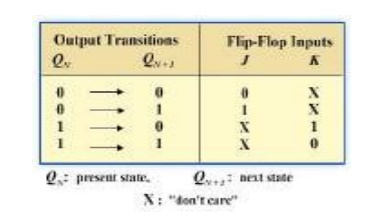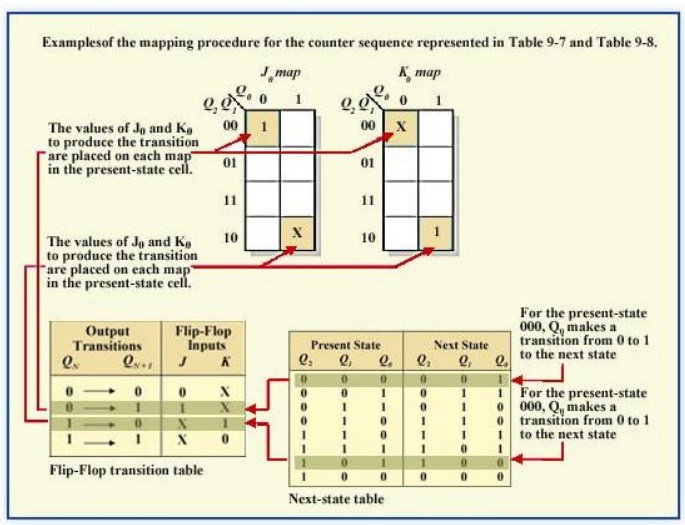Home | | Digital Electronics | Design of Synchronous Counters

# Design of Synchronous Counters

This section begins our study of designing an important class of clocked sequential logic circuits-synchronous finite-state machines.

DESIGN OF SYNCHRONOUS COUNTERS:

This section begins our study of designing an important class of clocked sequential logic circuits-synchronous finite-state machines. Like all sequential circuits, a finite-state machine determines its outputs and its next state from its current inputs and current state. A synchronous finite state machine changes state only on the clocking event.

## COUNTER DESIGN PROCEDURE:

Describe a general sequential circuit in terms of its basic parts and its input and outputs. Develop a state diagram for a given sequence. Develop a next-state table for a specific counter sequence. Create a FF transition table. Use K-map to derive the logic equations. Implement a counter to produce a specified sequence of states.

## Design the 3-bit Gray code counter

Step 1: State Diagram

State Diagram for a 3-bit Gray code counter:Step 2: Next-State Table

Next state table for a 3-bit Gray code counterStep 3: Flip-Flop Transition Table

Transition table for a J-K Flip-FlopStep 4: Karnaugh Maps

The following diagram shows the steps to create separate next states of separate J and K from the current states of J and K.Karnaugh maps for present-state J and K inputs for the 3-bit Gray code counter..Step 5: Logic Expressions for Flip-flop Inputs

The next-state J and K outputs for a 3-bit Gray code counter.Step 6: Counter Implementation

The hardware diagram of the 3-bit Gray code counter

1.    Design – Example 1

Design a counter with the irregular binary count sequence shown in the state diagram of Figure 4.1.

Step 1: State DiagramStep 2: Next-State TableStep 3: Flip-Flop Transition Table

Transition table for a J-K Flip-FlopStep 4: Karnaugh MapsStep 5: Logic Expressions for Flip-flop Inputs

The expression for each J and K input taken from the maps is as follows:

J0 = 1, K0 = Q2

J1 = K1 = 1

J2 = K2 = Q1

Step 6: Counter ImplementationExample 2 - Design the 3 Up/down counter (Gray code sequence)

Step 1: State DiagramStep 2: Next-State TableStep 3: Flip-Flop Transition Table

Transition table for a J-K Flip-FlopHere X denotes the don’t care condition Qn       is the present state of transition output and

Qn+1  is the next state of the transition output

Step 4: Karnaugh MapsStep 5: Logic Expressions for Flip-flop InputsStep 6: Counter ImplementationStudy Material, Lecturing Notes, Assignment, Reference, Wiki description explanation, brief detail
Digital Electronics : Sequential Circuits : Design of Synchronous Counters |